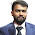Frank Hertz Expriment

Frank Hertz Expriment

Aim

To study Frank Hertz Experiment

Apparatus

Frank -Hertz Experiment setup model no FH3001.

Theory

The Frank Hertz tube in this instrument is a tetrode filled with the vapour of the experimental substance. The figure indicates the basic scheme of the experiment. The electrons emitted by filament can be accelerated by the potential `V_{G2K}` between the cathode and the grid G2. The grid G1 helps in minimizing space charge effects. The grids are wire mesh and allow the electrons to pass through. The plate A is maintained at a potential slightly negative with respect to grid G2. This helps in making the dips in the plate current more prominent. In this experiment, the electron current is measured as a function of the Voltage `V_{G2K}`.As the voltage increases the electron energy goes up and so the electron can overcome the retarding, potential `V_{G2A}` to reach the plate. This gives rise to a current in the ammeter, which initially increases. As the voltage further increases, the electron energy reaches the threshold value to excite the atom in its first allowed excited state. In doing so, the electron loose energy and therefore the number of electrons reaching the plate decreases. This decrease is proportional to the number of inelastic collisions that have occurred. When the `V_{G2K}` is increased further and reaches a value twice that of the first excitation potential, it is possible for an electron to excite an atom halfway between the grids, lose all its energy, and then gain a new enough energy to excite a second dip in the current. The advantage of this type of configuration of the potential is that the current dips are much more pronounced, and it is easy to obtain fivefold or larger multiplicity in the excitation of the first level.

1. Ensure that the Electrical power is 220 V,10,50 Hz.

2. Before the power is switched 'ON' make sure all the control knobs are at their minimum position and Current multiplier knob at 10-7 position.

3. Switch on the Manual auto switch to manual, and check that the scanning voltage knob is at its minimum position.

4. Turn the Voltage Display selector to `V_{G1K}` and adjust the `V_{G1K}` knob until voltmeter reads 1.5V.

5. Turn the Voltage Display selector to `V_{G1A}` and adjust the `V_{G2A}` knob until voltmeter read 7.5V

6. Before proceeding to the next step check that the initial parameters are
Filament Voltage: 2.6 V (minimum position)
`V_{G1K}` : 1.5  V
`V_{G2A}` : 7.5 V
`V_{G2K}` : 0  V
Current Amplifier `10^{-8}`

7. These are suggested values for the experiment. The experiment can be done with other values also.

8. Rotate `V_{G2K}` knob and observe the variation of plate current with the increase of `V_{G2K}`. The current reading should show maxima and minima periodically. The magnitude of maxima could be adjusted suitably by adjusting the filament voltage and the value of the current multiplier.

9. Now take the systematic reading, `V_{G2K}` vs Plate Current. For better resolution and observation of the maxima and minima, `V_{G2K}` is varied from 0-80 V in the increments of 0.1 V or 0.01 V. Increments of 0.1 will be used for the data set away from the peak or the dip. The interval 0.01 V may choose to finer the observation near maxima or minima.